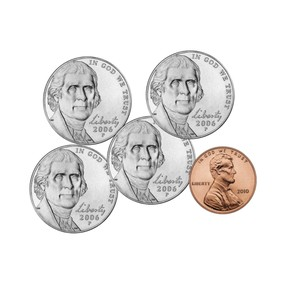Counting money to 100 cents

# Counting money to 100 cents

I can count money to 100 cents.8,000 schools use Gynzy92,000 teachers use Gynzy1,600,000 students use Gynzy

## General

Students will review the names and values of quarters, dimes, nickels, and pennies. They will practice counting combinations of these coins in a variety of activities.

## Standards

CCSS.Math.Content.2.MD.C.8

## Learning objective

Students will be able to count money to 100 cents.

## Introduction

Students will review skip counting by 5’s and 10’s. This will be related to the skip counting needed to count coin combinations.

## Instruction

Students are introduced to coins starting with the penny. They practice counting a group of pennies in order. Next, we move on to working with nickel. They review its value of 5 and then count groups of nickels. Following this, students practice finding the total of groups of nickels and pennies. They also complete an activity where they count a given amount of money. Students learn the value of a dime count dimes. To practice, they are shown a price tag with a corresponding group of coins, and they determine if the amount is correct. The final coin discussed is the quarter. They practice the skip counting sequence of 25, 50, 75, 100 by clapping along with it. Students count combinations with quarters by writing the numbers they say as they count. A dice-throwing game provides additional practice in counting with all 4 coins. The lesson then discusses how you can show coin amounts in different ways such as 2 nickels equals a dime. Instruction concludes with two story problems about money.

## Quiz

Students respond to ten multiple-choice and true/false questions.

## Closing

Students will arrange coins along a caterpillar’s back. They will count the coins and share the total.

### The online teaching platform for interactive whiteboards and displays in schools

• Save time building lessons

• Manage the classroom more efficiently

• Increase student engagement